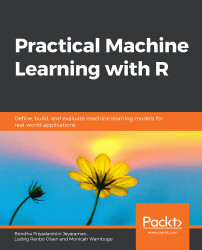•#### Practical Machine Learning with R#### Overview of this book

With huge amounts of data being generated every moment, businesses need applications that apply complex mathematical calculations to data repeatedly and at speed. With machine learning techniques and R, you can easily develop these kinds of applications in an efficient way. Practical Machine Learning with R begins by helping you grasp the basics of machine learning methods, while also highlighting how and why they work. You will understand how to get these algorithms to work in practice, rather than focusing on mathematical derivations. As you progress from one chapter to another, you will gain hands-on experience of building a machine learning solution in R. Next, using R packages such as rpart, random forest, and multiple imputation by chained equations (MICE), you will learn to implement algorithms including neural net classifier, decision trees, and linear and non-linear regression. As you progress through the book, you’ll delve into various machine learning techniques for both supervised and unsupervised learning approaches. In addition to this, you’ll gain insights into partitioning the datasets and mechanisms to evaluate the results from each model and be able to compare them. By the end of this book, you will have gained expertise in solving your business problems, starting by forming a good problem statement, selecting the most appropriate model to solve your problem, and then ensuring that you do not overtrain it.Free Chapter
An Introduction to Machine LearningData Cleaning and Pre-processingFeature EngineeringIntroduction to neuralnet and Evaluation MethodsLinear and Logistic Regression ModelsUnsupervised LearningAppendix## Model Selection by Multiple Disagreeing Metrics

What happens if the metrics do not agree on the ranking of our models? In the last chapter, on classification, we learned about the precision and recall metrics, which we "merged" into the F1 score, because it is easier to compare models on one metric than two. But what if we did not want to (or couldn't) merge two or more metrics into one (possibly arbitrary) metric?

### Pareto Dominance

If a model is better than another model on one metrics, and at least as good on all other metrics, this model should be considered better overall. We say that the model dominates the other model.

If we remove all the models that are dominated by other models, we will have the nondominated models left. This set of models is referred to as the Pareto set (or the Pareto front). We will see in a moment why Pareto front is a fitting name.

Let's say that our Pareto set consists of two models. One has high precision, but low recall. The other...